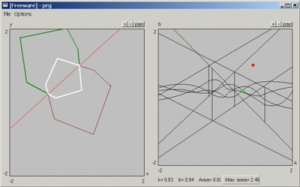# Maximizing the Area of an Axis-Symmetric Polygon Inscribed by a Simple Polygon

## Abstract

In this paper we solve the following optimization problem: Given a simple polygon P, what is the maximum-area polygon that is axially symmetric and is contained by P? We propose an algorithmfor solving this problem, analyze its complexity, and describe our implementation of it (for the case of a convex polygon). The algorithm is based on building and investigating a planar map, each cell of which corresponds to a different configuration of the inscribed polygon. We prove that the complexity of the map is O(n4), where n is the complexity of P. For a convex polygon the complexity is O(n3) in the worst case.

• Gill Barequet and Vadim Rogol
Maximizing the Area of an Axis-Symmetric Polygon Inscribed by a Simple Polygon [pdf]
• Gill Barequet and Vadim Rogol
Maximizing the Area of an Axis-Symmetric Polygon Inscribed by a Convex Polygon
The 4th Israel-Korea Bi-National Conference on Geometric Modeling and Computer Graphics. Conference Proceedings, 2003, 141-146.
[BibTex]